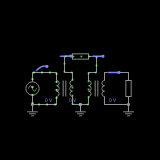thebugger modified 6 years ago

# How to determine an Unknown transformers parameters Extended221557305:37:39
In this example every value should be read as an RMS value even though it's the Peak value, for simplicity. Usually the [1.41][0.707] rule applies!!! Firstly I'd like to note that very often i find a transformer with no indication at all of what its parameters are. Here are the steps i use to determine the transformer's parameters. 1. It's not wise to directly supply an unknown transformer with 220V because you may not know which the primary is and which the secondary is. Moreover it could be an audio transformer which may burn it. So first you take a known transformer and step down the mains to a more controllable level. Let's say 16Vrms. 2. You measure the active DC resistance of the coils of the unknown transformer using an ohmmeter. Whichever has a higher resistance is the primary (that may not always apply but it's the case with step down transformers) 3. You connect the primary of the unknown transformer to the 16Vrms side of the known transformer and measure the voltage at the secondary of the unknown transformer. In our case it's 297mV. ⚠ Be careful because if you reverse the primary with the secondary you may end up stepping up the voltage to even above mains, which is lethal. Take great precaution in this step. 4. Now that we know the voltages of both the sides of the unknown transformer we can calculate its winding ratio. You just divide the primary voltage to the secondary. 16V/0.297V=54. Therefore the winding ratio is 54:1. Even though the transformer here says 50:10, with the active resistance dropping the voltage the ratio becomes 54:1 5. Now it's time to calculate the Primary Impedance. For this you must know the exact resistance of the load, because it will reflect to the primary's impedance. I will get to that in a sec. Our load is 1kohm. The formula is Rtsx(Wr)^2 [ohms], where Rts is the load (1k) and Wr is the winding ratio (54). So we get 1000x(50)^2. 1000x2500= 2916000ohms. So the primary impedance is 2.96Mohms. 6. The load at the secondary will reflect to the primary's impedance. For instance if we switch the load with a 500ohm resistor instead of a 1k one the formula takes this shape. 500x(54)^2=1458000ohm. Therefore the primary impedance has changed from 2.96Mohm to twice as low 1.46Mohm. This is called reflected impedance. 7. Now if you know the primary impedance and you want to determine the secondary the formula takes this shape. Rtp/(Wr)^2, where Rtp is the primary impedance in ohms. So 2916000/(54^2)=1000ohms. Therefore the secondary impedance is 1k. 8. Now, how to determine the primary inductance. First you have to measure the active DC resistance of the primary of the unknown transformer. Let's assume it's 125ohm. Typically all loads should be removed for a reliable reading. Now we must measure the current through the primary to calculate the reactive resistance of the coil. In our case it's 47mA. So R=U/I = 340ohm. Now we use the geometric addition formula Z^2=R^2+Xl^2. Now a little mathematical substitution and rationalizing and we get that Xl=sqrt(Z^2-R^2) X=sqrt (340^2-125^2)=316ohm. So the reactive resistance for the primary of the unknown transformer is 316ohm for 50Hz. Now that we know that, we must use the reactance formula Xl=2xПxfxL and substitute to find L=Xl/2пf L=340/6.28*50= 1.08H. As you can see it is really close to the true inductance in the example, but that's what you get for rounding numbers.
published 7 years ago
 2ctiby 7 years ago
 That's a handy article to keep around thanks.
 thebugger 7 years ago
 I like to have things written down in case i need it, and don't remember the formulas.
 agungpratamaputra 7 years ago
 Hh
 giomix 7 years ago
 Good how-to, usefull, often I discover we use the same or similar methods to know component's parameters.
 thebugger 7 years ago
 Engineering is all about practice, screw theory :D most discoveries, were made by accident, while experimenting.
 giomix 7 years ago
 I agree with you, curiosity and practice help to find something new
 colloquiallyineffable 7 years ago
 Sometimes, if there's enough space, you can thread a single turn of wire around your existing windings and use the voltage it develops to find the number of turns on each the factory windings. If that helps anyone.
 colloquiallyineffable 7 years ago
 When i was trying to figure out the phasing of a 120/240:14 125A xfmr i used just put a 40W lightbulb in series with my power so that if I screwed up, worst case, the bulb lights up.
 zorgrian 7 years ago
 Go look at Wikipedia - cut your lectures
 zorgrian 7 years ago
 I often discover that this toad needs therapy
 zorgrian 7 years ago
 Vote @thebugger for president of the USA! Pure genius !!!
 thebugger 7 years ago
 What's your fucking problem. If you don't want to read it, just dont. Cut the snappy comments you dork. You go to wikipedia and see if it's helpful about such things. I find it most unhelpful
 thebugger 7 years ago
 If you don't find this useful just don't look at it. I've made this for myself and for the people who find it helpful.
 GuyHardy 6 years ago